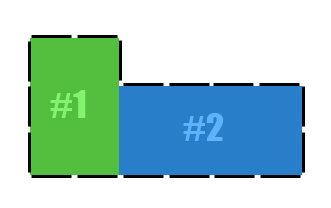# Sand Box L Shaped Volume Calculator

This calculator requires the use of Javascript enabled and capable browsers. This calculator will determine the approximate volume of sand required. It is for all configurations of (two) rectangle structures, such as a generally "L" shaped structure, as depicted in our graphic below. For calculation reasons and to create an "L" shaped structure, the number 1 rectangle must be larger vertically than the joining number 2 rectangle's joining width. Each rectangle forms a part of the "L". The number 1 rectangle is the vertical side of the "L", while number 2 is the horizontal base of the "L".

In this example and calculator, for both rectangles, enter the number of inches for each width side... Enter the number of inches for the length side; see our default information and graphic below. (Please keep in mind that the width of the number 2 rectangle joins into number 1 vertical length, though not the TOTAL length.) Also enter the number of inches high each side is (8 inches by default); and press the Calculate button. (Each rectangle's height can be different to compensate for sloped ground.) The approximate volume of filling material is in cubic inches, cubic feet and cubic yards for your convenience.Designation Value Required Data Entry First Rectangle (Vertical of L) Inches Per End Width Side (1 or more) Horizontal Top of L Inches Per Length (Opposite of Joining) Side (join to #2) Inches High Second Rectangle (Base Side of L) Inches Per Width (Joining) Side (join to #1 - 1 or more) Inches Per (perpendicular) Length Side (1 or more) Inches High (1 or more) Total Structure Volume (cubic inches) Total Structure Volume (cubic feet) Total Structure Volume (cubic yards) Volume #1 (cubic inches) Volume #2 (cubic inches)

Version 1.1.1

 Leave us a question or comment on FacebookSearch or Browse Our Site